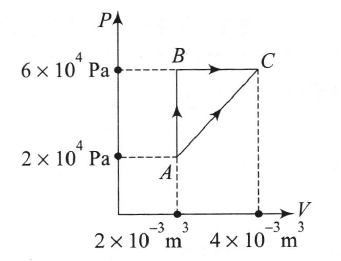Figure below shows two paths that may be taken by a gas to go from a state A to a state C. In process AB, 400 J of heat is added to the system and in process BC, 100 J of heat is added to the system. The heat absorbed by the system in the process AC will be

# Figure below shows two paths that may be taken by a gas to go from a state A to a state C. In process AB, 400 J of heat is added to the system and in process BC, 100 J of heat is added to the system. The heat absorbed by the system in the process AC will be1. A

380 J

2. B

500 J

3. C

460 J

4. D

300 J

Register to Get Free Mock Test and Study Material

+91

Verify OTP Code (required)

### Solution:

In cyclic process

400 + l00 + ${\mathrm{Q}}_{\mathrm{CA}}$ = $\frac{1}{2}×\left(4×{10}^{4}×2×{10}^{-3}\right)$Register to Get Free Mock Test and Study Material

+91

Verify OTP Code (required)